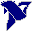# LabVIEW

cancel
Showing results for
Did you mean:

Solved!
Go to solution

## Re: How to calculate the equation from dataset using "Fitting VI's" (polynomial or nonlinear Fit) ?

Everything is ok,except for the fact, that now you saying that is "recommended to convert from resistance to temperature (and not the other way around).... But why is that? I've been previously being doing this and everything seems ok... So why the recommendation, I don't get it... Is it the predicted equation less accurate??

Regards,

Message 21 of 25
(683 Views)

## Re: How to calculate the equation from dataset using "Fitting VI's" (polynomial or nonlinear Fit) ?

Hi JLuna,

is "recommended to convert from resistance to temperature (and not the other way around).... But why is that?

Usually you measure the resistance of your thermistor and want to calculate the temperature from this value…

Best regards,
GerdWusing LV2011SP1 + LV2017 (+LV2020 sometimes) on Win10+cRIO
Message 22 of 25
(681 Views)

## Re: How to calculate the equation from dataset using "Fitting VI's" (polynomial or nonlinear Fit) ?

The reason I came up with this problem is because:

• Based on a detected temperature I need to be in certain levels of NTC resistance. So my question is, if I use the original structure, been the Resistance values - "x" and the respective temperature values - "f(x)" .... Is there an inconvinience with the calculated Polynomial Fit Curve Equation...??
Message 23 of 25
(670 Views)

## Re: How to calculate the equation from dataset using "Fitting VI's" (polynomial or nonlinear Fit) ?

The polynomial solution should work well  both ways.

(Programming the inverse Stainhart-Hart equation is a bit more tricky).LabVIEW Champion. It all comes together inGCentralWhat does "Engineering Redefined" mean??
Message 24 of 25
(667 Views)

## Re: How to calculate the equation from dataset using "Fitting VI's" (polynomial or nonlinear Fit) ?

Sir. Many thanks for your help, I really appreciate it!!

Best regards!

Message 25 of 25
(646 Views)